# 基于分区径向基函数配点法的大变形分析1)

*同济大学航空航天与力学学院, 上海 200092
†上海宇航系统工程研究所, 上海 201109

# FINTE SUBDOMAIN RADIAL BASIS COLLOCATION METHOD FOR THE LARGE DEFORMATION ANALYSIS1)

Wang Lihua*2), Li Yiming*, Chu Fuyun

*School of Aerospace Engineering and Applied Mechanics， Tongji University， Shanghai 200092， China
†Aerospace System Engineering Shanghai， Shanghai 201109， China

Abstract

The meshfree methods can avoid grid distortion problems because it does not need to be meshed, which make them widely used in large deformations and other complicated problems. Radial basis collocation method (RBCM) is a typical strong form meshfree method. This method has the advantages of no need for any mesh, simple solution process, high precision, good convergence and easy expansion to high-dimensional problems. Since the global shape function is used, this method has the disadvantages of low precision and poor representation to local characteristics when solving high gradient problems. To resolve this issue, this paper introduces finite subdomain radial basis collocation method to solve the large deformation problem with local high gradients. Based on the total Lagrangian formulation, the Newton iteration method is used to establish the incremental solution scheme of the FSRBCM in large deformation analysis. This method partitions the solution domain into several subdomains according to its geometric characteristics, then constructs radial basis function interpolation in the subdomains, and imposes all the interface continuous conditions on the interfaces, which results in a block sparse matrix for the numerical solution. The proposed method has super convergence and transforms the original full matrix into a sparse matrix, which reduces the storage space and improves the computational efficiency. Compared to the traditional RBCM and finite element method (FEM), this method can better reflect local characteristics and solve high gradient problems. Numerical simulations show that the method can effectively solve the large deformation problems with local high gradients.

Keywords： meshfree ; finite subdomain radial basis collocation method ; large deformation ; high gradient ; Newton iteration method

0

Wang Lihua, Li Yiming, Chu Fuyun. FINTE SUBDOMAIN RADIAL BASIS COLLOCATION METHOD FOR THE LARGE DEFORMATION ANALYSIS1)[J]. Chinese Journal of Theoretical and Applied Mechanics, 2019, 51(3): 743-753 https://doi.org/10.6052/0459-1879-19-005

## 1 径向基函数近似

$$g_I \left( {\pmb x} \right) = \left( {r_I^2 + c^2} \right)^{\vartheta - \tfrac{3}{2}} (1)$$

## 2 大变形分析

### 2.1 基本方程

$$\left.\begin{array}{ll} \left( {S_{kj} F_{ik} } \right)_{,j} + b_i = {0} \,, & {\rm in} \ \varOmega S_{jk} F_{ij} N_k = h_i & {\rm on} \ \varGamma u_i = g_i\,, & {\rm on} \ \varPi \end{array} \right \} (2)$$

$$S_{ij} = D_{ijkl} \varepsilon _{kl} (3)$$

$$\varepsilon _{ij} = \dfrac{1}{2}\left( {\dfrac{\partial u_i }{\partial X_j } + \dfrac{\partial u_j }{\partial X_i } + \dfrac{\partial u_k }{\partial X_i}\dfrac{\partial u_k }{\partial X_j }} \right) (4)$$

$$\left.\begin{array}{ll} \left( {S_{kj}^\alpha F_{ik}^\alpha } \right)_{,j} + b_i^\alpha = {0}\,, & {\rm in} \ \varOmega ^\alpha S_{jk}^\alpha F_{ij}^\alpha N_k^\alpha = h_i^\alpha \,, & {\rm in} \ \varGamma ^\alpha u_i^\alpha = g_i^\alpha \,, & {\rm in} \ \varPi ^\alpha \end{array} \right \} (5)$$

$$\left.\begin{array}{l} S_{jk}^\alpha F_{ij}^\alpha N_k^\alpha + S_{jk}^\beta F_{ij}^\beta N_k^\beta = 0 u_i ^\alpha = u_i ^\beta \end{array} \right\} \quad {\rm in} \ \varLambda _{\alpha \beta } (6)$$

### 2.2 近似函数

$$\left( {u^\alpha \left( {\pmb X} \right)} \right)^h = \sum_{I = 1}^{\xi ^\alpha } {g_I^\alpha \left( {\pmb X} \right)a_I } ,\quad X \in \bar {\varOmega }^\alpha (7)$$

$$\left( {{u}_i^\alpha } \right)^h = \left( {{\pmb \varPhi }^\alpha } \right)^{\rm T}{\pmb a} (8)$$

$$\left( {{\pmb \varPhi }^\alpha } \right)^{\rm T} = \left[ {{\pmb g}_1^\alpha ,{\pmb g}_{2}^\alpha , \cdots ,{\pmb g}_{N^\alpha }^\alpha } \right]\,,\quad {\pmb g}_I^\alpha = g_I^\alpha {\pmb I} (9)$$

$$\left.\!\! {\pmb P}^\alpha = \{{\pmb p}_I \}_{I = 1}^{\zeta _p^\alpha } \subseteq \varOmega ^\alpha \,,\quad {\pmb Q}^\alpha = \{{\pmb q}_I \}_{I = 1}^{\zeta _q^\alpha } \subseteq \varGamma ^\alpha {\pmb R}^\alpha = \{{\pmb r}_I \}_{I = 1}^{\zeta _r^\alpha } \subseteq \varPi ^\alpha ,\quad {\pmb Z}_{\alpha \beta } = \{{\pmb z}_I \}_{I = 1}^{\zeta _{\alpha \beta } } \subseteq \varLambda _{\alpha \beta } \!\!\right\} (10)$$

### 2.3 大变形问题求解

$$\left.\!\!\begin{array}{ll} \Delta \left[ {\left( {S_{kj}^\alpha F_{ik}^\alpha } \right)_{,j} + b_i^\alpha } \right]_{v + 1}^n = - \left[ {\left( {S_{kj}^\alpha F_{ik}^\alpha } \right)_{,j} + b_i^\alpha } \right]_v^n \,, & {\rm in} \ \varOmega ^\alpha \Delta \left( {S_{kj}^\alpha F_{ik}^\alpha N_j^\alpha } \right)_{v + 1}^n = \left( {h_i^\alpha } \right)^n - \left( {S_{kj}^\alpha F_{ik}^\alpha N_j^\alpha } \right)_v^n \,, & {\rm in} \ \varGamma ^\alpha \Delta \left( {u_i^\alpha } \right)_{v + 1}^n = \left( {g_i^\alpha } \right)^n - \left( {u_i^\alpha } \right)_v^n \,, & {\rm in} \ \varPi ^\alpha \end{array} \!\! \right \} (11)$$

$\left( {F_{ik,j}^\alpha } \right)_v \left( {\Delta S_{kj}^\alpha } \right)_{v + 1} + \left( {S_{kj}^\alpha } \right)_v \left( {\Delta F_{ik,j}^\alpha } \right)_{v + 1} + \left( {F_{ik}^\alpha } \right)_v \left( {\Delta S_{kj,j}^\alpha } \right)_{v + 1} + \left( {S_{kj,j}^\alpha } \right)_v \left( {\Delta F_{ik}^\alpha } \right)_{v + 1}= b_i^\alpha - \left( {F_{ik,j}^\alpha S_{kj}^\alpha + F_{ik}^\alpha S_{kj,j}^\alpha } \right)_v \,, \ \ {\rm in} \ \varOmega ^\alpha (12)$

$\left( {S_{kj}^\alpha N_k^\alpha } \right)_v \left( {\Delta F_{ik}^\alpha } \right)_{v + 1} + \left( {F_{ik}^\alpha N_j^\alpha } \right)_v \left( {\Delta S_{kj}^\alpha } \right)_{v + 1} = h_i^\alpha - \left( {S_{kj}^\alpha F_{ik}^\alpha N_j^\alpha } \right)_v \,, \ \ {\rm in} \ \varGamma ^\alpha (13)$

$\left( {\Delta u_i^\alpha } \right)_{v + 1} = g_i^\alpha - \left( {u_i^\alpha } \right)_v \,, \ \ {\rm in} \ \varPi ^\alpha (14)$

$\left( {S_{kj}^\alpha N_j^\alpha } \right)_v \left( {\Delta F_{ik}^\alpha } \right)_{v + 1} + \left( {F_{ik}^\alpha N_j^\alpha } \right)_v \left( {\Delta S_{kj}^\alpha } \right)_{v + 1} +$

$\qquad \left( {S_{kj}^\beta N_j^\beta } \right)_v \left( {\Delta F_{ik}^\beta } \right)_{v + 1} + \left( {F_{ik}^\beta N_j^\beta } \right)_v \left( {\Delta S_{kj}^\beta } \right)_{v + 1} =$

$\qquad - \left( {S_{kj}^\alpha F_{ik}^\alpha N_j^\alpha } \right)_v - \left( {S_{kj}^\beta F_{ik}^\beta N_j^\beta } \right)_v \,, \ \ {\rm in} \ \varLambda _{\alpha \beta } (15)$

$\left( {\Delta u_i^\alpha } \right)_{v + 1} - \left( {\Delta u_i^\beta } \right)_{v + 1} = \left( {u_i^\beta } \right)_v - \left( {u_i^\alpha } \right)_v \,, \ \ {\rm in} \ \varLambda _{\alpha \beta } (16)$

$${\pmb K} \Delta {\pmb a} = {\pmb f} (17)$$

${\pmb K} = \left[\!\!\begin{array}{c} {{\pmb K}^1} {{\pmb K}^2} \vdots {{\pmb K}^m} \end{array} \!\!\right]\,,\ {\pmb K}^\alpha = \left[ \!\!\begin{array}{c} {{\pmb K}_L^\alpha } {{\pmb K}_h^\alpha } {{\pmb K}_g^\alpha } {{\pmb K}_{\Lambda _h }^\alpha } {{\pmb K}_{\Lambda _g }^\alpha } \end{array} \!\! \right]\,, \ {\pmb f} = \left[\!\!\begin{array}{c} {{\pmb f}^1} {{\pmb f}^2} \vdots {{\pmb f}^m} \end{array} \!\! \right]\,,\ {\pmb f}^\alpha = \left[ \!\!\begin{array}{c} {{\pmb f}_L^\alpha } {{\pmb f}_h^\alpha } {{\pmb f}_g^\alpha } {{\pmb f}_{\Lambda _h }^\alpha } {{\pmb f}_{\Lambda _g }^\alpha } \end{array} \!\! \right] (18)$

${\pmb K}_L^\alpha = {\pmb K}_L^\alpha = {\pmb L \pmb D}_1 {\pmb B}_1 + \left( {{\pmb L}_2 {\pmb D}_2 {\pmb A}_3 + {\pmb S}_1 } \right){\pmb B}_2 +$

$\qquad \left( {{\pmb L\pmb D}_1 {\pmb A}_1 + {\pmb L}_2 {\pmb D}_2 {\pmb A}_2 + {\pmb T}_1 + {\pmb T}_2 } \right){\pmb B}_3 (19)$

${\pmb K}_h^\alpha = {\pmb K}_{\Lambda _h }^\alpha = {\pmb L}_2 {\pmb N}_1 {\pmb D}_1 ({\pmb B}_1 + {\pmb A}_1 {\pmb B}_3 ) + {\pmb N}_2 {\pmb S}_2 {\pmb B}_3 (20)$

${\pmb K}_g^\alpha = {\pmb K}_{\varLambda _g }^\alpha = \left[ \!\!\begin{array}{cc} \left( {{\pmb \varPhi }^\alpha } \right)^{\rm T} & {\bf 0} {\bf 0} & \left( {{\pmb \varPhi }^\alpha } \right)^{\rm T} \end{array} \!\! \right] (21)$

${\pmb f}_L^\alpha = {\pmb b} - {\pmb L\pmb S} - {\pmb L}_2 \left( {{\pmb T}_3 + {\pmb T}_4 } \right) (22)$

${\pmb f}_h^\alpha = {\pmb h} - {\pmb N}_2 {\pmb L}_3 {\pmb S} (23)$

${\pmb f}_g^\alpha = {\pmb g} - {\pmb u} (24)$

${\pmb f}_{\varLambda _h }^\alpha = {\pmb f}_{\varLambda _g }^\alpha = {\bf 0} (25)$

$${\pmb W \pmb K} \Delta {\pmb a} = {\pmb W \pmb f} (26)$$

$${\pmb W} = \left[\!\!\begin{array}{c} {{\pmb W}^1} {{\pmb W}^2} \vdots {{\pmb W}^m} \end{array} \!\! \right]\,\,,\quad {\pmb W}^\alpha = \left[\!\! \begin{array}{c} {\pmb 1} {{\pmb W}_h^\alpha } {{\pmb W}_g^\alpha } {{\pmb W}_{\varLambda _h }^\alpha } {{\pmb W}_{\varLambda _g }^\alpha } \end{array}\!\! \right] (27)$$

$$\left. w_h^\alpha \approx O\left( {\theta ^\alpha } \right)\,, \ \ w_g^\alpha \approx O\left( {\kappa ^\alpha \xi ^\alpha } \right) w_{\Lambda _h }^{\alpha \beta } \approx O\left( 1 \right)\,, \ \ w_{\Lambda _g }^{\alpha \beta } \approx O\left( {\kappa ^{\alpha \beta }\xi ^{\alpha \beta }} \right) \!\!\right\} (28)$$

a其中,$\kappa ^\alpha = \max \left( {\lambda ^\alpha ,\mu ^\alpha } \right)$, $\kappa ^{\alpha \beta } = \max(\kappa ^\alpha ,\kappa ^\beta )$, $\theta ^\alpha = \kappa ^{\alpha \beta } / \kappa ^\alpha$, $\xi^{\alpha \beta } = \max (\xi ^\alpha ,\xi ^\beta )$, $\xi ^\alpha$和 $\xi ^\beta$即对应$\alpha$和$\beta$两个子域内选取的源点数目, $\lambda ^\alpha$和$\mu ^\alpha$为$\alpha$子域中材料的拉梅常数.超定方程(27)通常可以采用最小二乘法、截断奇异值分解法等方法进行求解.

### 2.4 程序设计

(1)确定初始构型, 区域划分模式,子域个数以及域内、边界和界面上的源点和配点分布, 设置载荷增量.

(2)在第一个载荷步中(在第$n$个载荷步中), 取第一个迭代步的初始近似值${\pmb a}_0$.

(3)计算得到${\pmb u}_0 = {\pmb \varPhi \pmb a}_0$(${\pmb u}_v = {\pmb \varPhi \pmb a}_v$). 根据式(19) $\sim$式(25)和${\pmb u}_0$ (${\pmb u}_v$)计算出每个子域上的${\pmb K}_L$, ${\pmb K}_h$, ${\pmb K}_g$, ${\pmb K}_{\varLambda _h }$, ${\pmb K}_{\varLambda _g }$, ${\pmb f}_L$, ${\pmb f}_h$, ${\pmb f}_g$, ${\pmb f}_{\varLambda _h }$, ${\pmb f}_{\varLambda _h }$, 组装成整体矩阵${\pmb K}$和${\pmb f}$, 通过方程(17)求出增量$\Delta {\pmb a}_1$ ($\Delta {\pmb a}_v$).

(4)判断$\Delta {\pmb u}_1 = {\pmb \varPhi }\Delta {\pmb a}_1$ ($\Delta {\pmb u}_v = {\pmb \varPhi }\Delta {\pmb a}_v$)是否满足下式

$$\left\| {\Delta {\pmb u}} \right\| \leqslant e (29)$$

$${\pmb a}_1 = {\pmb a}_0 + \Delta {\pmb a}_1 \;\left( { {\pmb a}_{v + 1} = {\pmb a}_v + \Delta {\pmb a}_{v + 1} } \right) (30)$$

(5)在载荷中增加载荷增量进入下一个分析步.然后依据式(2) $\sim$式(4)的过程循环求解, 直至载荷步全部计算结束.

## 3 数值算例

### 3.1 受均布载荷作用的悬臂梁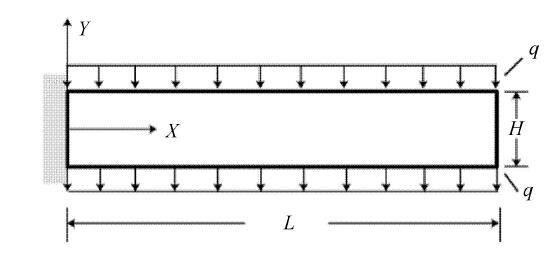Fig. 1   Cantivilever beam under uniform load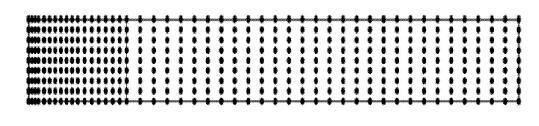Fig. 2   Distribution of source points for cantivilever beam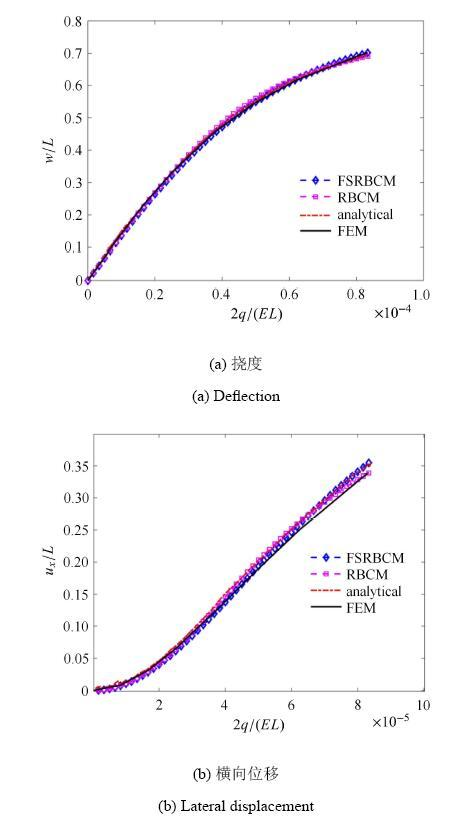Fig. 3   Deflection and lateral displacement of the end point of cantilever beam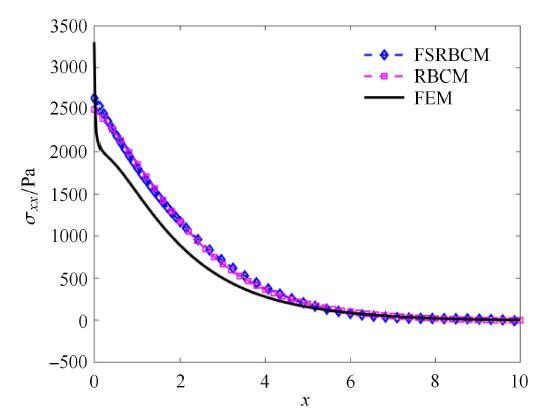Fig. 4   Cauchy stress on the upper surface of cantilever beam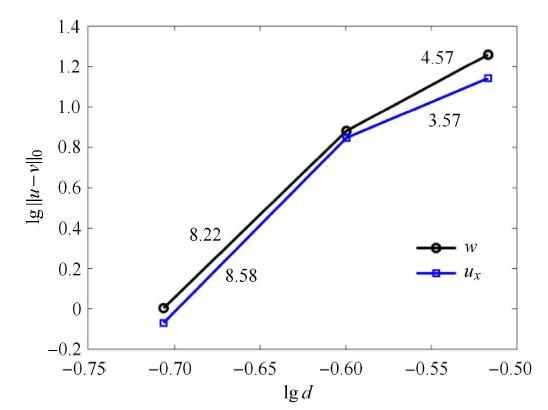Fig. 5   Convergence of the deflection and lateral displacement for cantilever beam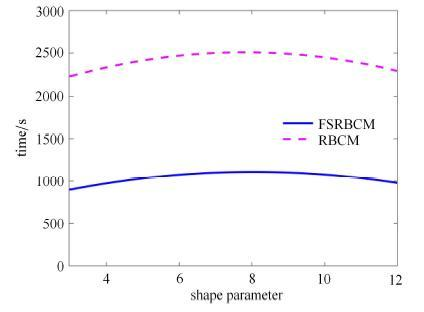Fig. 6   Comparison of the CPU time for cantilever beam

### 3.2 纯弯曲悬臂梁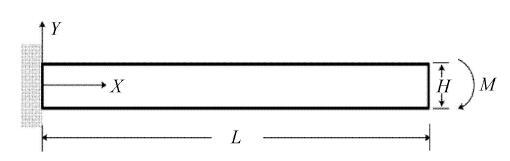Fig. 7   Cantivilever beam under bending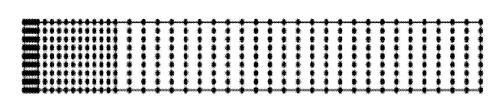Fig. 8   Distribution of source points for pure bending beam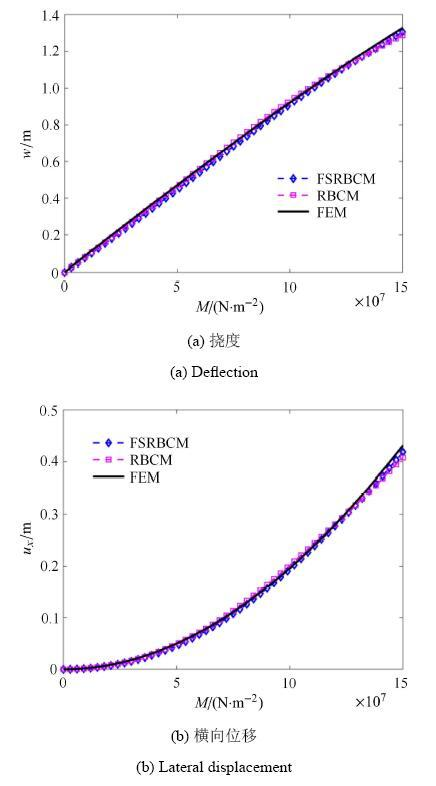Fig. 9   Deflection and lateral displacement of the end point of pure bending beam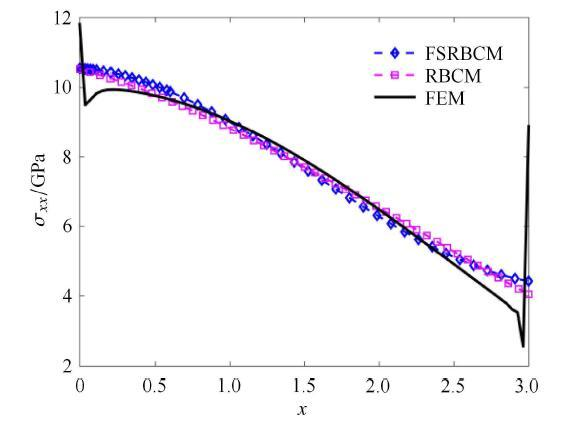Fig. 10   Cauchy stress on the upper surface of pure bending beam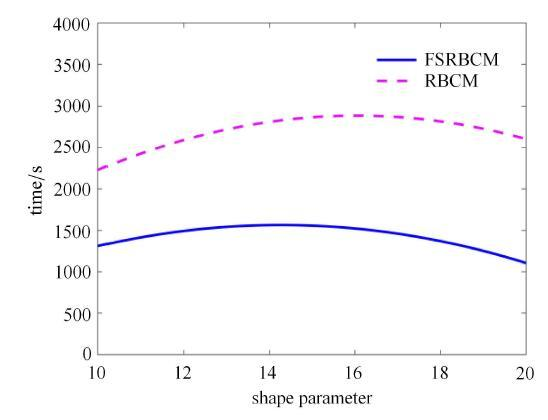Fig. 11   Comparison of CPU time for pure bending beam

### 3.3 拉伸作用下的带孔板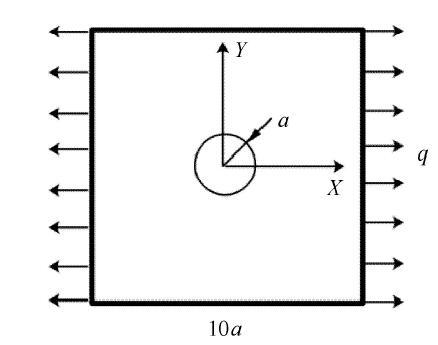Fig. 12   Plate with hole under tension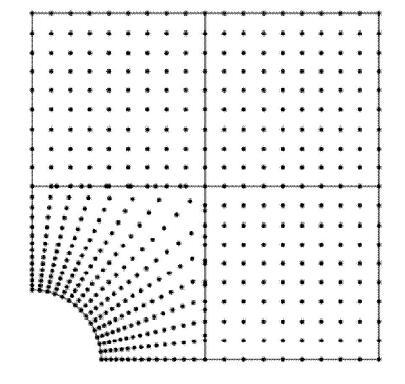Fig. 13   Distribution of source points for plate with holeFig. 14   Displacement of the simply supported boundary of plate with hole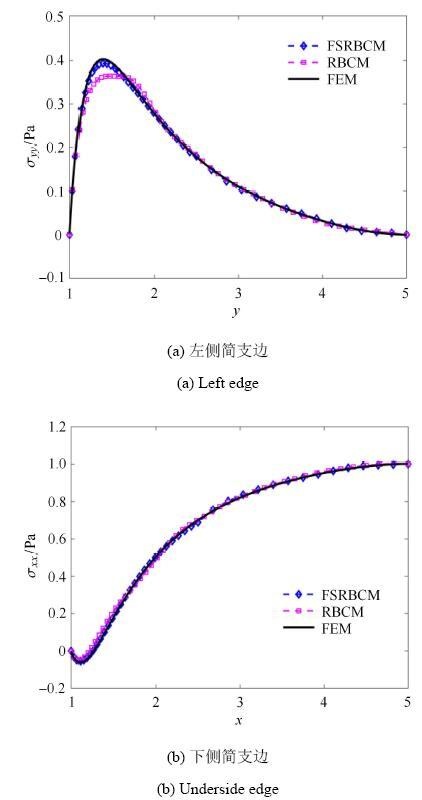Fig. 15   Cauchy stress of the simply supported boundary of plate with hole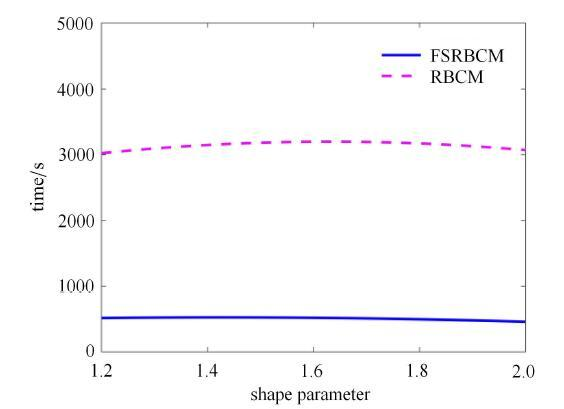Fig. 16   Comparison of CPU time for plate with hole

## 附录The authors have declared that no competing interests exist.

## 参考文献 原文顺序 文献年度倒序 文中引用次数倒序 被引期刊影响因子〈〉